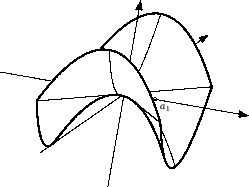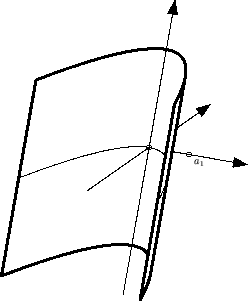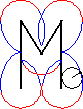[home] [lexicon] [problems] [tests] [courses] [auxiliaries] [notes] [staff]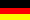Mathematics-Online lexicon:

# Euclidean Normal Forms of Three-Dimensional Quadrics

 A B C D E F G H I J K L M N O P Q R S T U V W X Y Z overview

There exist appropriate Cartesian coordinate systems with respect to which the equations defining quadrics have the following normal forms.

 normal form name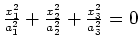point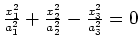(double) cone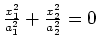line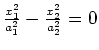intersecting planes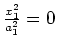coincident planes

 normal form name(empty set)hyperboloid of 2 sheets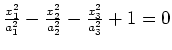hyperboloid of 1 sheetellipsoid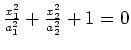(empty set)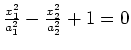hyperbolic cylinder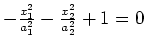elliptic cylinder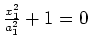(empty set)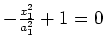parallel planes

 normal form nameelliptic paraboloid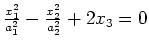hyperbolic paraboloid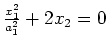parabolic cylinder

The normal forms are uniquely determined up to permutation of subscripts and in the case of conical quadrics up to multiplication by a constant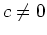.

The values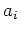are set to be positive and are called lengths of the principal axes of the quadric.

 (double) cone intersecting planes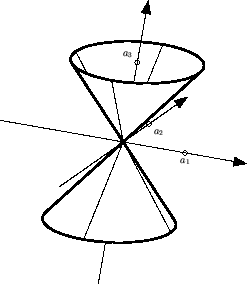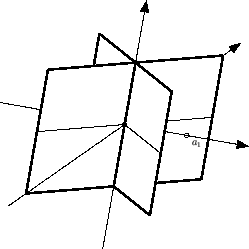hyperboloid of 2 sheets hyperboloid of 1 sheet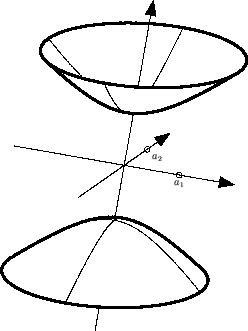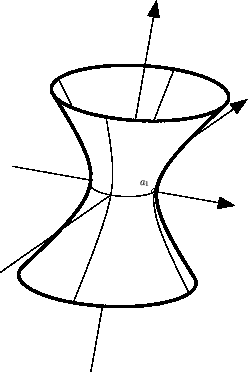ellipsoid hyperbolic cylinder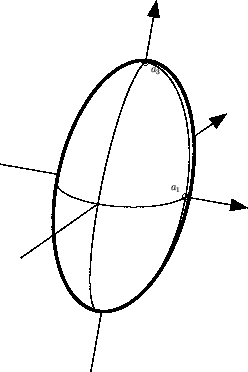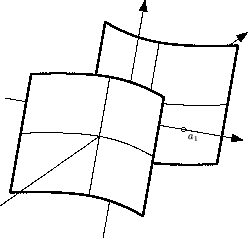elliptic cylinder elliptic paraboloid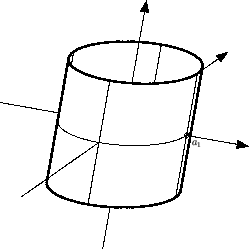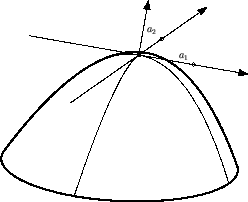hyperbolic paraboloid parabolic cylinder